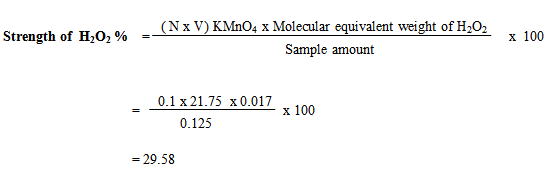# How to Determine the Strength of Hydrogen Peroxide (H2O2)

Last Updated on 09/01/2021

### How to Determine the Strength of Hydrogen Peroxide (H2O2)

Mustaque Ahammed Mamun
Department of Textile Engineering
Dhaka University of Engineering & Technology (DUET)
Email: mamuntex09@gmail.com

Introduction:
Hydrogen Peroxide (H2O2) is widely used as bleaching agent in textile industry. It is used for the bleaching of natural, vegetable and animal fibers derived from cellulose such as cotton, linen and other bast fibers. Among the oxidizing agents Hydrogen Peroxide (H2O2) has high bleaching effect at reasonable cost. It keeps fiber quality good. In this article I will determine strength of Hydrogen Peroxide (H2O2).

The strength of H2O2 is expressed in two ways-

1. Volumetric
2. Percentage (%w/v)

Volumetric: In this way, the strength is expressed as the volume of oxygen gas liberated from one unit of H2O2 liquor under standard condition (i.e. N.T.P.)

For example: If 1cc of H2O2 liberates 100cc oxygen at S.T.P. The strength of H2O2 will be called 100 volumes H2O2.

Percentage (%w/v): This is the weight of H2O2 per unit volume. This is more commonly used in our country.

Generally 35% H2O2 and 50% H2O2 used in textile sector in our country. But 50% H2O2 is most widely used. If 50 gm H2O2 is present in 100cc solution, then it will be called 50% H2O2.

Objectives:

1. To know about how determined the strength of H2O2.
2. To know about the titration process.
3. To know about the solution making.
4. To know about the end point of this testing.

Apparatus:

1. Beaker
2. Pipette
3. Stirrer
5. Electronic balance

Reagents:

1. 0.1 N KMnO4
2. 10 % H2SO4
3. And H2O2

Procedure:

2.5 ml H2O2 is taken

Then it is diluted with 500 ml water

From that solution 25 ml is taken

After that it is diluted with 25 ml 10% H2SO4

Then it is taken in Conical flask. Then this sample solution is treated with 0.1 N KMnO4

End Point: The end point is determined when the colorless solution becomes permanent pink in color.

Calculation:

2KMnO4 + 3H2SO4 + 5H2O2 → K2SO4 + 2MnSO4 + 8H2O +5O2

Molecular equivalent weight of H2O2 = 17 gm

So, 1000 ml Molecular equivalent weight is 17

1 ml molecular equivalent weight is = 17/1000 = 0.017 gm

In 500 ml water the amount of sample (H2O2) is 2.5 ml

So, 25 ml of water the amount of sample (H2O2) is = (2.5 x 25) / 500 = 0.125

Normality of KMnO4 is 0.1 N

And volume used of KMnO4 is 21.75

Following formula is used for determine strength of Hydrogen Peroxide:Results and discussion:

 Required Amount of KMnO4 Strength of H2O2 21.75 29.58% 22 29.92% 22.1 30.056% 21.80 29.648% 20.3 27.608%

From above results, we can say that the strength of H2O2 is near as 30% which was indicated at the bottle but our different persons results have been obtained near to 30% and my results is 29.58% which is less than 30% because we have done experiment manually so something can be inaccurate measurement of pipette or burette reading. But our last person results is 27.608% which is very less than 30%, so I think that, his experiment more inaccurate measurement of different chemicals.

Conclusion:
At last we can say that, how to determine the strength of H2O2, is known by this experiment. Knowledge is gained from this experiment which is very helpful in our practical life.